GATE  >  Practice Test: Gate Civil Engineering(CE) 2014 Paper

# Practice Test: Gate Civil Engineering(CE) 2014 Paper

Test Description

## 65 Questions MCQ Test GATE Past Year Papers for Practice (All Branches) | Practice Test: Gate Civil Engineering(CE) 2014 Paper

Practice Test: Gate Civil Engineering(CE) 2014 Paper for GATE 2023 is part of GATE Past Year Papers for Practice (All Branches) preparation. The Practice Test: Gate Civil Engineering(CE) 2014 Paper questions and answers have been prepared according to the GATE exam syllabus.The Practice Test: Gate Civil Engineering(CE) 2014 Paper MCQs are made for GATE 2023 Exam. Find important definitions, questions, notes, meanings, examples, exercises, MCQs and online tests for Practice Test: Gate Civil Engineering(CE) 2014 Paper below.
Solutions of Practice Test: Gate Civil Engineering(CE) 2014 Paper questions in English are available as part of our GATE Past Year Papers for Practice (All Branches) for GATE & Practice Test: Gate Civil Engineering(CE) 2014 Paper solutions in Hindi for GATE Past Year Papers for Practice (All Branches) course. Download more important topics, notes, lectures and mock test series for GATE Exam by signing up for free. Attempt Practice Test: Gate Civil Engineering(CE) 2014 Paper | 65 questions in 180 minutes | Mock test for GATE preparation | Free important questions MCQ to study GATE Past Year Papers for Practice (All Branches) for GATE Exam | Download free PDF with solutions
 1 Crore+ students have signed up on EduRev. Have you?
Practice Test: Gate Civil Engineering(CE) 2014 Paper - Question 1

### Q. 1 – Q. 5 carry one mark each. A student is required to demonstrate a high level of comprehension of the subject, especially in the social sciences. The word closest in meaning to comprehension is

Detailed Solution for Practice Test: Gate Civil Engineering(CE) 2014 Paper - Question 1

Comprehension means the ability to understand something.
E.g. Some people won’t be able to comprehend the project on which I am working.
This means that some people won’t be able to understand the project on which I am working.

Practice Test: Gate Civil Engineering(CE) 2014 Paper - Question 2

### Choose the most appropriate word from the options given below to complete the following sentence. One of his biggest ______ was his ability to forgive.

Detailed Solution for Practice Test: Gate Civil Engineering(CE) 2014 Paper - Question 2

Virtue is moral excellence. A virtue is a trait or quality that is deemed to be morally good and thus is valued as a foundation of principle and good moral being. Thus, the statement will be- One of the biggest virtues was his ability to forgive.

Practice Test: Gate Civil Engineering(CE) 2014 Paper - Question 3

### Rajan was not happy that Sajan decided to do the project on his own. On observing his unhappiness, Sajan explained to Rajan that he preferred to work independently. Which one of the statements below is logically valid and can be inferred from the above sentences?

Practice Test: Gate Civil Engineering(CE) 2014 Paper - Question 4

If y = 5x2 + 3, then the tangent at x = 0, y = 3

Detailed Solution for Practice Test: Gate Civil Engineering(CE) 2014 Paper - Question 4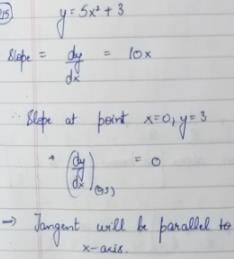*Answer can only contain numeric values
Practice Test: Gate Civil Engineering(CE) 2014 Paper - Question 5

A foundry has a fixed daily cost of Rs 50,000 whenever it operates and a variable cost of Rs 800Q, where Q is the daily production in tonnes. What is the cost of production in Rs per tonne for a daily production of 100 tonnes?

Detailed Solution for Practice Test: Gate Civil Engineering(CE) 2014 Paper - Question 5

Fixed Cost= Rs. 50000
Daily Production = 100 tonnes
Variable Cost = Rs 800Q = Rs 800 x 100 = Rs 80000
Total Cost = Fixed Cost + Variable Cost = Rs 50000+ Rs 80000= Rs 130000
Therefore, Cost of Production per tonne= Rs 130000/100 tonnes= Rs 1300/ tonne

Practice Test: Gate Civil Engineering(CE) 2014 Paper - Question 6

Q. 6 – Q. 10 carry two marks each.

Find the odd one in the following group: ALRVX, EPVZB, ITZDF, OYEIK

Detailed Solution for Practice Test: Gate Civil Engineering(CE) 2014 Paper - Question 6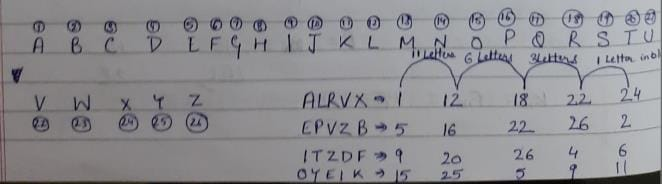Practice Test: Gate Civil Engineering(CE) 2014 Paper - Question 7

Anuj, Bhola, Chandan, Dilip, Eswar and Faisal live on different floors in a six-storeyed building (the ground floor is numbered 1, the floor above it 2, and so on). Anuj lives on an even-numbered floor. Bhola does not live on an odd numbered floor. Chandan does not live on any of the floors below Faisal’s floor. Dilip does not live on floor number 2. Eswar does not live on a floor immediately above or immediately below Bhola. Faisal lives three floors above Dilip. Which of the following floor-person combinations is correct?

*Answer can only contain numeric values
Practice Test: Gate Civil Engineering(CE) 2014 Paper - Question 8

The smallest angle of a triangle is equal to two thirds of the smallest angle of a quadrilateral. The ratio between the angles of the quadrilateral is 3:4:5:6. The largest angle of the triangle is twice its smallest angle. What is the sum, in degrees, of the second largest angle of the triangle and the largest angle of the quadrilateral?

Detailed Solution for Practice Test: Gate Civil Engineering(CE) 2014 Paper - Question 8

Let angle of quadrilateral be 3x, 4x, 5x, 6x
Sum of angles of quadrilateral = 360 = 3x+4x+5x+6x = 18x
x= 360/18 =20
The smallest angle of triangle is ⅔ of smallest angle of quadrilateral = ⅔ (3x)
= 2x = 40
Largest angle of triangle is twice the smallest angle of triangle= 2x40 = 80
Therefore, second largest angle of triangle = 180-Largest Angle- Smallest Angle
= 180 - 80 -40 = 60
Sum of second largest triangle and largest angle of quadrilateral = 60+ 6x
= 60+ 6x20
=180

Practice Test: Gate Civil Engineering(CE) 2014 Paper - Question 9

One percent of the people of country X are taller than 6 ft. Two percent of the people of country Y are taller than 6 ft. There are thrice as many people in country X as in country Y. Taking both countries together, what is the percentage of people taller than 6 ft?

Practice Test: Gate Civil Engineering(CE) 2014 Paper - Question 10

The monthly rainfall chart based on 50 years of rainfall in Agra is shown in the following figure. Which of the following are true? (k percentile is the value such that k percent of the data fall below that value)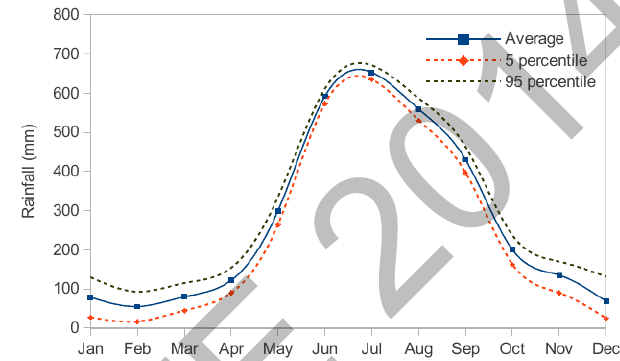(i) On average, it rains more in July than in December
(ii) Every year, the amount of rainfall in August is more than that in January
(iii) July rainfall can be estimated with better confidence than February rainfall
(iv) In August, there is at least 500 mm of rainfall

Practice Test: Gate Civil Engineering(CE) 2014 Paper - Question 11

Q. 11 – Q. 35 carry one mark each.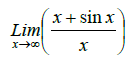equals to

Detailed Solution for Practice Test: Gate Civil Engineering(CE) 2014 Paper - Question 11

Separate the two fractions → 1+ (sinx)/x
It is the property of the sin function that its values remain in between -1 and 1. However, the denominator value of x is approaching infinity.
Therefore, (sinx)/x when x approaches infinity will be equal to zero.
Thus, the value of the limit equals 1.

*Answer can only contain numeric values
Practice Test: Gate Civil Engineering(CE) 2014 Paper - Question 12

Given the matrices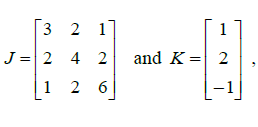the product KT JK is ___________

Detailed Solution for Practice Test: Gate Civil Engineering(CE) 2014 Paper - Question 12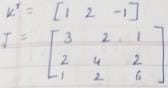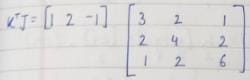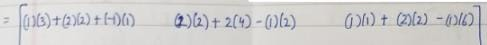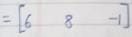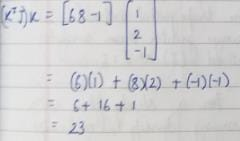*Answer can only contain numeric values
Practice Test: Gate Civil Engineering(CE) 2014 Paper - Question 13

The probability density function of evaporation E on any day during a year in a watershed is given by

Practice Test: Gate Civil Engineering(CE) 2014 Paper - Question 14

The sum of Eigen values of the matrix, [M] is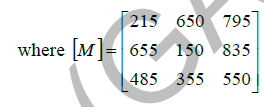Detailed Solution for Practice Test: Gate Civil Engineering(CE) 2014 Paper - Question 14

Sum of Eigen Values = Sum of Diagonal Elements of Matrix = 215+150+550 = 915

Practice Test: Gate Civil Engineering(CE) 2014 Paper - Question 15

With reference to the conventional Cartesian (x, y) coordinate system, the vertices of a triangle have the following coordinates: (x1, y1) = (1, 0); (x2, y2) = (2, 2); and (x3, y3) = (4, 3). The area of the triangle is equal to

Practice Test: Gate Civil Engineering(CE) 2014 Paper - Question 16

Match the information given in Group – I with those in Group - II.

Group – I                                                                             Group – II
P Factor to decrease ultimate strength to                          1 Upper bound on ultimate load
design strength

Q Factor to increase working load to                                  2 Lower bound on ultimate load

R Statical method of ultimate load                                     3 Material partial safety factor
analysis

S Kinematical mechanism method of                                 4 Load factor

Practice Test: Gate Civil Engineering(CE) 2014 Paper - Question 17

The possible location of shear centre of the channel section, shown below, is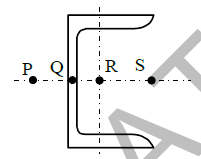Detailed Solution for Practice Test: Gate Civil Engineering(CE) 2014 Paper - Question 17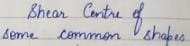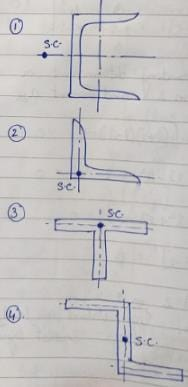Practice Test: Gate Civil Engineering(CE) 2014 Paper - Question 18

The ultimate collapse load (P) in terms of plastic moment Mp by kinematic approach for a propped cantilever of length L with P acting at its mid-span as shown in the figure, would be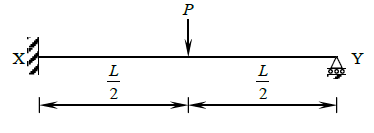*Answer can only contain numeric values
Practice Test: Gate Civil Engineering(CE) 2014 Paper - Question 19

While designing, for a steel column of Fe250 grade, a base plate resting on a concrete pedestal of M20 grade, the bearing strength of concrete (in N/mm2) in limit state method of design as per IS:456-2000 is ________________

Practice Test: Gate Civil Engineering(CE) 2014 Paper - Question 20

A steel section is subjected to a combination of shear and bending actions. The applied shear force is V and the shear capacity of the section is Vs. For such a section, high shear force (as per IS:800- 2007) is defined as

Practice Test: Gate Civil Engineering(CE) 2014 Paper - Question 21

The degree of static indeterminacy of a rigid jointed frame PQR supported as shown in the figure is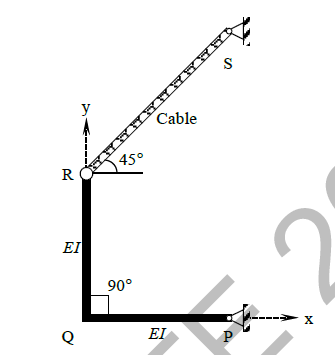Practice Test: Gate Civil Engineering(CE) 2014 Paper - Question 22

In a beam of length L, four possible influence line diagrams for shear force at a section located at a distance of L/4 from the left end support (marked as P, Q, R and S) are shown below. The correct influence line diagram is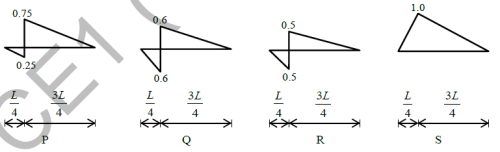Practice Test: Gate Civil Engineering(CE) 2014 Paper - Question 23

The degree of disturbance of the sample collected by the sampler is expressed by a term called the "area ratio". If the outer diameter and inner diameter of the sampler are Do and Di respectively, the area ratio is given by

Practice Test: Gate Civil Engineering(CE) 2014 Paper - Question 24

For a saturated cohesive soil, a triaxial test yields the angle of internal friction (φ) as zero. The conducted test is

Practice Test: Gate Civil Engineering(CE) 2014 Paper - Question 25

The action of negative skin friction on the pile is to

Practice Test: Gate Civil Engineering(CE) 2014 Paper - Question 26

A long slope is formed in a soil with shear strength parameters: c' = 0 and φ' = 34°. A firm stratum lies below the slope and it is assumed that the water table may occasionally rise to the surface, with seepage taking place parallel to the slope. Use γsat = 18 kN/m3 and γw = 10 kN/m3. The maximum slope angle (in degrees) to ensure a factor of safety of 1.5, assuming a potential failure surface parallel to the slope, would be

*Answer can only contain numeric values
Practice Test: Gate Civil Engineering(CE) 2014 Paper - Question 27

An incompressible homogeneous fluid is flowing steadily in a variable diameter pipe having the large and small diameters as 15 cm and 5 cm, respectively. If the velocity at a section at the 15 cm diameter portion of the pipe is 2.5 m/s, the velocity of the fluid (in m/s) at a section falling in 5 cm portion of the pipe is ___________

Practice Test: Gate Civil Engineering(CE) 2014 Paper - Question 28

A conventional flow duration curve is a plot between

Practice Test: Gate Civil Engineering(CE) 2014 Paper - Question 29

In reservoirs with an uncontrolled spillway, the peak of the plotted outflow hydrograph

Detailed Solution for Practice Test: Gate Civil Engineering(CE) 2014 Paper - Question 29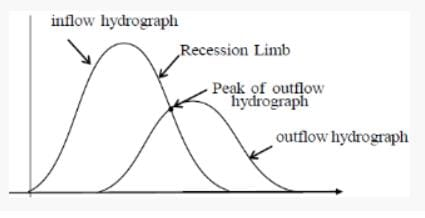Practice Test: Gate Civil Engineering(CE) 2014 Paper - Question 30

The dimension for kinematic viscosity is

Practice Test: Gate Civil Engineering(CE) 2014 Paper - Question 31

Some of the nontoxic metals normally found in natural water are

*Answer can only contain numeric values
Practice Test: Gate Civil Engineering(CE) 2014 Paper - Question 32

The amount of CO2 generated (in kg) while completely oxidizing one kg of CH4 to the end products is ____________

Practice Test: Gate Civil Engineering(CE) 2014 Paper - Question 33

The minimum value of 15 minute peak hour factor on a section of a road is

Practice Test: Gate Civil Engineering(CE) 2014 Paper - Question 34

The following statements are related to temperature stresses developed in concrete pavement slabs with free edges (without any restraint):

P. The temperature stresses will be zero during both day and night times if the pavement slab is considered weightless
Q. The temperature stresses will be compressive at the bottom of the slab during night time if the self-weight of the pavement slab is considered
R. The temperature stresses will be compressive at the bottom of the slab during day time if the self-weight of the pavement slab is considered

The TRUE statement(s) is(are)

Detailed Solution for Practice Test: Gate Civil Engineering(CE) 2014 Paper - Question 34

If slab in considered weightless it will result in its E value=0, thus, no temperature stresses.
If slab weight considered, during day the upper part of slab will be heated due to sunlight in comparison to lower slab. This leads to compressive stress at top and tensile stress at bottom. Exactly opposite that is tensile at top and compressive at bottom occurs during night.
Format:Textual
Question number – 193
Forum Id – 609559
Solution: (a)
For dilution coefficient equal to 1
Weight of chlorine to be used/(square of discharge) = constant
32/(16*16) = x / (22*22)
x=60.5 kg/day

Practice Test: Gate Civil Engineering(CE) 2014 Paper - Question 35

The Reduced Levels (RLs) of the points P and Q are +49.600 m and +51.870 m respectively. Distance PQ is 20 m. The distance (in m from P) at which the +51.000 m contour cuts the line PQ is

Practice Test: Gate Civil Engineering(CE) 2014 Paper - Question 36

Q. 36 – Q. 65 carry two marks each.

If the following equation establishes equilibrium in slightly bent position, the mid-span deflection of a member shown in the figure is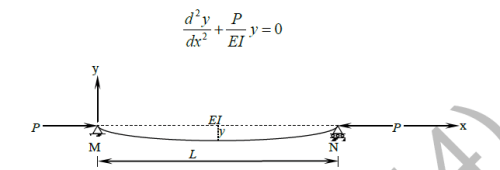If a is amplitude constant for y, then

Practice Test: Gate Civil Engineering(CE) 2014 Paper - Question 37

A box of weight 100 kN shown in the figure is to be lifted without swinging. If all forces are coplanar, the magnitude and direction (θ) of the force (F) with respect to x-axis should be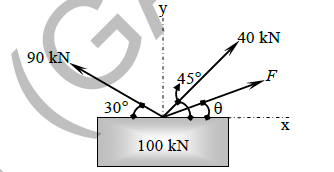Detailed Solution for Practice Test: Gate Civil Engineering(CE) 2014 Paper - Question 37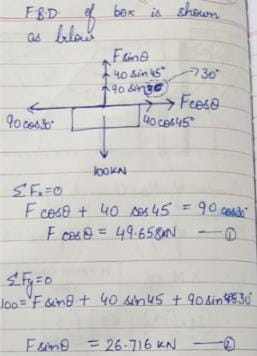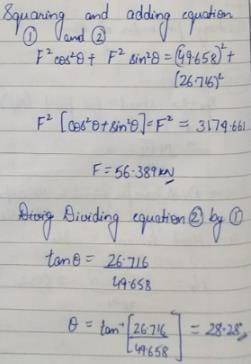*Answer can only contain numeric values
Practice Test: Gate Civil Engineering(CE) 2014 Paper - Question 38

A particle moves along a curve whose parametric equations are: x = t3 + 2t, y = −3e−2t and z = 2 sin (5t), where x, y and z show variations of the distance covered by the particle (in cm) with time t (in s). The magnitude of the acceleration of the particle (in cm/s2) at t = 0 is ________

*Answer can only contain numeric values
Practice Test: Gate Civil Engineering(CE) 2014 Paper - Question 39

A traffic office imposes on an average 5 number of penalties daily on traffic violators. Assume that the number of penalties on different days is independent and follows a Poisson distribution. The probability that there will be less than 4 penalties in a day is ___________

Practice Test: Gate Civil Engineering(CE) 2014 Paper - Question 40

Mathematical idealization of a crane has three bars with their vertices arranged as shown in the figure with a load of 80 kN hanging vertically. The coordinates of the vertices are given in parentheses. The force in the member QR, FQR will be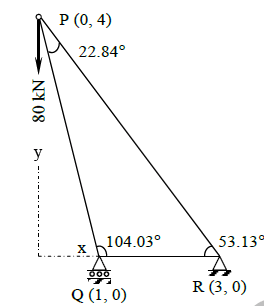*Answer can only contain numeric values
Practice Test: Gate Civil Engineering(CE) 2014 Paper - Question 41

For the cantilever beam of span 3 m (shown below), a concentrated load of 20 kN applied at the free end causes a vertical displacement of 2 mm at a section located at a distance of 1 m from the fixed end. If a concentrated vertically downward load of 10 kN is applied at the section located at a distance of 1 m from the fixed end (with no other load on the beam), the maximum vertical displacement in the same beam (in mm) is __________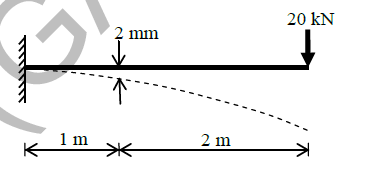*Answer can only contain numeric values
Practice Test: Gate Civil Engineering(CE) 2014 Paper - Question 42

For the truss shown below, the member PQ is short by 3 mm. The magnitude of vertical displacement of joint R (in mm) is _______________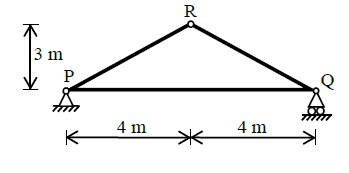Practice Test: Gate Civil Engineering(CE) 2014 Paper - Question 43

A rectangular beam of width (b) 230 mm and effective depth (d) 450 mm is reinforced with four bars of 12 mm diameter. The grade of concrete is M20 and grade of steel is Fe500. Given that for M20 grade of concrete the ultimate shear strength, τuc = 0.36 N/mm2 for steel percentage, p = 0.25, and τuc = 0.48 N/mm2 for p = 0.50. For a factored shear force of 45 kN, the diameter (in mm) of Fe500 steel two legged stirrups to be used at spacing of 375 mm, should be

Practice Test: Gate Civil Engineering(CE) 2014 Paper - Question 44

The tension and shear force (both in kN) in each bolt of the joint, as shown below, respectively are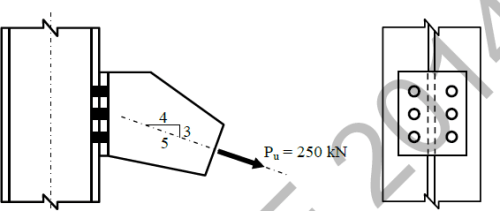*Answer can only contain numeric values
Practice Test: Gate Civil Engineering(CE) 2014 Paper - Question 45

For a beam of cross-section, width = 230 mm and effective depth = 500 mm, the number of rebars of 12 mm diameter required to satisfy minimum tension reinforcement requirement specified by IS:456-2000 (assuming grade of steel reinforcement as Fe500) is _____________

Practice Test: Gate Civil Engineering(CE) 2014 Paper - Question 46

In a reinforced concrete section, the stress at the extreme fibre in compression is 5.80 MPa. The depth of neutral axis in the section is 58 mm and the grade of concrete is M25. Assuming linear elastic behavior of the concrete, the effective curvature of the section (in per mm) is

Practice Test: Gate Civil Engineering(CE) 2014 Paper - Question 47

Group I contains representative load-settlement curves for different modes of bearing capacity failures of sandy soil. Group II enlists the various failure characteristics. Match the load-settlement curves with the corresponding failure characteristics.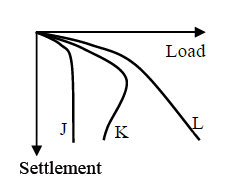Group I                                                  Group II
P. Curve J                                              1. No apparent heaving of soil around the footing
Q. Curve K                                             2. Rankine’s passive zone develops imperfectly
R. Curve L                                              3. Well defined slip surface extends to ground surface

Practice Test: Gate Civil Engineering(CE) 2014 Paper - Question 48

A given cohesionless soil has emax = 0.85 and emin = 0.50. In the field, the soil is compacted to a mass density of 1800 kg/m3 at a water content of 8%. Take the mass density of water as 1000 kg/m3 and Gs as 2.7. The relative density (in %) of the soil is

*Answer can only contain numeric values
Practice Test: Gate Civil Engineering(CE) 2014 Paper - Question 49

The following data are given for the laboratory sample.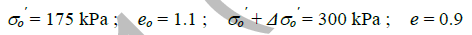If thickness of the clay specimen is 25 mm, the value of coefficient of volume compressibility is ___________ ×10-4 m2/kN

*Answer can only contain numeric values
Practice Test: Gate Civil Engineering(CE) 2014 Paper - Question 50

The flow net constructed for the dam is shown in the figure below. Taking the coefficient of permeability as 3.8×10−6 m/s, the quantity of flow (in cm3/s) under the dam per meter of dam is ______________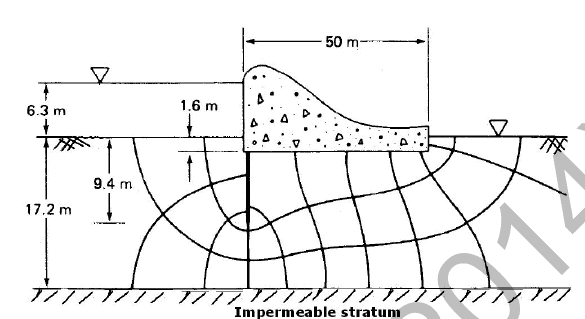Detailed Solution for Practice Test: Gate Civil Engineering(CE) 2014 Paper - Question 50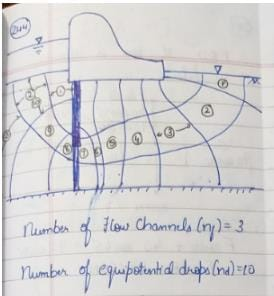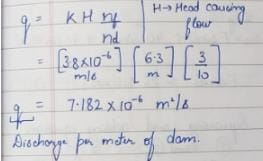Practice Test: Gate Civil Engineering(CE) 2014 Paper - Question 51

A horizontal jet of water with its cross-sectional area of 0.0028 m2 hits a fixed vertical plate with a velocity of 5 m/s. After impact the jet splits symmetrically in a plane parallel to the plane of the plate. The force of impact (in N) of the jet on the plate is

*Answer can only contain numeric values
Practice Test: Gate Civil Engineering(CE) 2014 Paper - Question 52

A venturimeter, having a diameter of 7.5 cm at the throat and 15 cm at the enlarged end, is installed in a horizontal pipeline of 15 cm diameter. The pipe carries an incompressible fluid at a steady rate of 30 litres per second. The difference of pressure head measured in terms of the moving fluid in between the enlarged and the throat of the venturimeter is observed to be 2.45 m. Taking the acceleration due to gravity as 9.81 m/s2, the coefficient of discharge of the venturimeter (correct up to two places of decimal) is ______________

Practice Test: Gate Civil Engineering(CE) 2014 Paper - Question 53

A rectangular channel having a bed slope of 0.0001, width 3.0 m and Manning’s coefficient ‘n’ 0.015, carries a discharge of 1.0 m3/s. Given that the normal depth of flow ranges between 0.76 m and 0.8 m. The minimum width of a throat (in m) that is possible at a given section, while ensuring that the prevailing normal depth is not exceeded along the reach upstream of the contraction, is approximately equal to (assume negligible losses)

Practice Test: Gate Civil Engineering(CE) 2014 Paper - Question 54

Three rigid buckets, shown as in the figures (1), (2) and (3), are of identical heights and base areas. Further, assume that each of these buckets have negligible mass and are full of water. The weights of water in these buckets are denoted as W1, W2, and W3 respectively. Also, let the force of water on the base of the bucket be denoted as F1, F2, and F3 respectively. The option giving an accurate description of the system physics is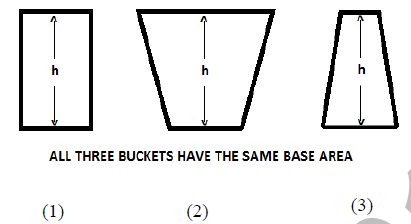*Answer can only contain numeric values
Practice Test: Gate Civil Engineering(CE) 2014 Paper - Question 55

An incompressible fluid is flowing at a steady rate in a horizontal pipe. From a section, the pipe divides into two horizontal parallel pipes of diameters d1 and d2 (where d1 = 4d2) that run for a distance of L each and then again join back to a pipe of the original size. For both the parallel pipes, assume the head loss due to friction only and the Darcy-Weisbach friction factor to be the same. The velocity ratio between the bigger and the smaller branched pipes is _________

Practice Test: Gate Civil Engineering(CE) 2014 Paper - Question 56

16 MLD of water is flowing through a 2.5 km long pipe of diameter 45 cm. The chlorine at the rate of 32 kg/d is applied at the entry of this pipe so that disinfected water is obtained at the exit. There is a proposal to increase the flow through this pipe to 22 MLD from 16 MLD. Assume the dilution coefficient, n = 1. The minimum amount of chlorine (in kg per day) to be applied to achieve the same degree of disinfection for the enhanced flow is

Detailed Solution for Practice Test: Gate Civil Engineering(CE) 2014 Paper - Question 56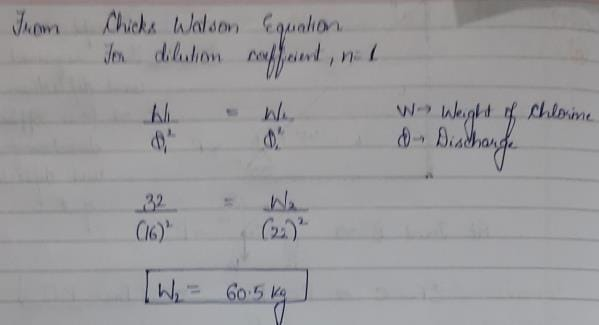Practice Test: Gate Civil Engineering(CE) 2014 Paper - Question 57

The potable water is prepared from turbid surface water by adopting the following treatment sequence.

Practice Test: Gate Civil Engineering(CE) 2014 Paper - Question 58

For a sample of water with the ionic composition shown in the figure below, the carbonate and noncarbonate hardness concentrations (in mg/l as CaCO3), respectively are: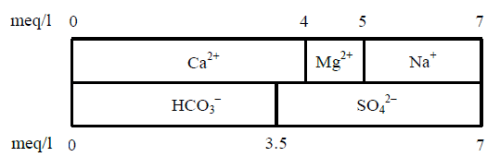*Answer can only contain numeric values
Practice Test: Gate Civil Engineering(CE) 2014 Paper - Question 59

A straight 100 m long raw water gravity main is to carry water from an intake structure to the jack well of a water treatment plant. The required flow through this water main is 0.21 m3/s. Allowable velocity through the main is 0.75 m/s. Assume f = 0.01, g = 9.81 m/s2. The minimum gradient (in cm/100 m length) to be given to this gravity main so that the required amount of water flows without any difficulty is ___________

*Answer can only contain numeric values
Practice Test: Gate Civil Engineering(CE) 2014 Paper - Question 60

A traffic survey conducted on a road yields an average daily traffic count of 5000 vehicles. The axle load distribution on the same road is given in the following table: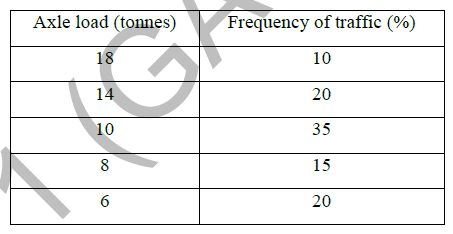The design peroid of the road is 15 years, the yearly traffic growth rate is 7.5% and the load safety
factor (LSF) is 1.3. If the vehicle damage factor (VDF) is calculated from the above data, the design
traffic (in million standard axle load, MSA) is ____________

*Answer can only contain numeric values
Practice Test: Gate Civil Engineering(CE) 2014 Paper - Question 61

The perception-reaction time for a vehicle travelling at 90 km/h, given the coefficient of longitudinal friction of 0.35 and the stopping sight distance of 170 m (assume g = 9.81 m/s2), is _____________ seconds.

*Answer can only contain numeric values
Practice Test: Gate Civil Engineering(CE) 2014 Paper - Question 62

The speed-density (u−k) relationship on a single lane road with unidirectional flow is u = 70 − 0.7k, where u is in km/hr and k is in veh/km. The capacity of the road (in veh/hr) is ___________

*Answer can only contain numeric values
Practice Test: Gate Civil Engineering(CE) 2014 Paper - Question 63

An isolated three-phase traffic signal is designed by Webster's method. The critical flow ratios for three phases are 0.20, 0.30, and 0.25 respectively, and lost time per phase is 4 seconds. The optimum cycle length (in seconds) is ___________

Practice Test: Gate Civil Engineering(CE) 2014 Paper - Question 64

A levelling is carried out to establish the Reduced Levels (RL) of point R with respect to the Bench Mark (BM) at P. The staff readings taken are given below.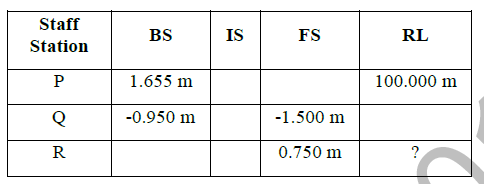If RL of P is +100.000 m, then RL (in m) of R is

Practice Test: Gate Civil Engineering(CE) 2014 Paper - Question 65

Group I lists tool/instrument while Group II lists the method of surveying. Match the tool/instrument with the corresponding method of surveying.

Group I                                      Group II
Q. Arrow                                   2. Levelling
R. Bubble tube                         3. Plain table surveying
S. Stadia hair                           4. Theodolite surveying

## GATE Past Year Papers for Practice (All Branches)

407 docs|127 tests
 Use Code STAYHOME200 and get INR 200 additional OFF Use Coupon Code
Information about Practice Test: Gate Civil Engineering(CE) 2014 Paper Page
In this test you can find the Exam questions for Practice Test: Gate Civil Engineering(CE) 2014 Paper solved & explained in the simplest way possible. Besides giving Questions and answers for Practice Test: Gate Civil Engineering(CE) 2014 Paper, EduRev gives you an ample number of Online tests for practice

## GATE Past Year Papers for Practice (All Branches)

407 docs|127 tests